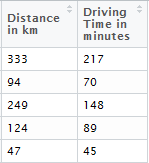Want to share your content on R-bloggers? click here if you have a blog, or here if you don't.

Geo-Distances are of great importance: Researchers from various disciplines refer to geographic distances – health researchers refer to geographic data when analyzing the spread of diseases, economists when evaluating the impact of transaction costs on human behavior, or sociologists when evaluating interpersonal distances (based on external factors) in human interaction.

However, each query sent to the Google Maps Distance Matrix API (currently available via the ggmap-package) is limited by the number of allowed elements, where the number of origins times the number of destinations defines the number of elements. The Google Maps Distance Matrix API has the following limits in place (Users of the standard API):

• 2,500 free elements per day
• 100 elements per query
• 100 elements per 10 seconds

Thus, researchers face a limit in requesting distances. This code proposes a work-around, respectively, an approach to request the distances; specifically, the proposed code requests the driving distance and driving time between two geographical points via google maps without any API restrictions. However, the code is quiet flexible and could be adjusted to request line-distances, etc.

The example refers to the attached csv-file. The comments are part of the script.

Example csv-file

You need five R packages (data.tablehttr, stringr, XML) to run the code.

Remarks, hints and further modifications are welcome.

In the first step, you have to load the relevant packages and the attached data files, which consists of four lot/lan distances.

```library("data.table")
library("httr")
library("stringr")
library("XML")

Second, define the URL codes to request the distances via google maps.

```newdata\$URL <- with(newdata, paste("https://www.google.de/maps/dir/",lat1,"+",lon1,"/",lat2,",",lon2, sep=""))
newdata\$URL <- as.character(newdata\$URL)```

```# Function Extracting the last n characters from a string
substrRight <- function(x, n){
substr(x, nchar(x)-n+1, nchar(x))
}
#######################################################################
# Function to request google maps driving distance
cnamet <- as.data.table(as.character(GET(url)))
cnamet <- as.character(cnamet)
# Compute Distance
dis<-substring((strsplit(substrRight(strsplit(cnamet,"km")[], 9), ",")[]), 2)
dis

# Compute Time
# Minutes
dur_m <- as.numeric(gsub( "[^[:alnum:],]", "", substrRight(strsplit(cnamet,"Min.")[], 4) ))
dur_m

# Hours (if applicable)
durh_h_new<-as.numeric(gsub( "[^[:alnum:]]", "",
ifelse(grepl("Std", substrRight(strsplit(cnamet,"Min")[], 15))=="TRUE",
str_extract_all(substrRight(strsplit(substrRight(strsplit(cnamet,"Std")[], 3),"Std.")[], 5),"\(?[0-9,.]+\)?")[],
"0")))
durh_h_new
# Change in Minutes
dur_fin<-dur_m+(durh_h_new*60)
dur_fin
# Combine all
fin<- as.character(paste (dis, dur_fin, sep = " ", collapse = NULL))
fin
}```

Finally, run the corresponding function for your data (here: example data set).

```# First Row: Google URL
# Second Column: Distance
# Third Column: Driving Time (Hint: Always the current driving time . might differ due traffic!!!)
colnames(files) <- "Distance in km"
colnames(files) <- "Driving Time in minutes"```

That’s it. Now, you should get the following output data file.The post Google Geo Data – Data Access Without Restrictions appeared first on ThinkToStart.